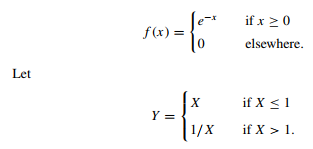### Create an Account

Home / Questions / Let X be a random variable with the probability density function given by Find the probabi...

# Let X be a random variable with the probability density function given by Find the probability density function of

Let X be a random variable with the probability density function given byFind the probability density function of Y .

Aug 02 2020 View more View LessSubscribe To Get Solution